Samacheer Kalvi 6th Maths Solutions Term 1 Chapter 5 Statistics Ex 5.1Tamilnadu Samacheer Kalvi 6th Maths Solutions Term 1 Chapter 5 Statistics Ex 5.1

Question 1.
Fill in the blanks.
(i) The collected information is called _____
(ii) An example of Primary data is ______
(iii) An example of a Secondary data is ______
(iv) The tally marks for number 8 in standard form is _____
Solution:
(i) Data
(ii) List of Absentees in a class
(iii) Cricket scores gathered from a website
(iv)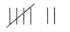Question 2.
Viji threw a die 30 times and noted down the result each time as follows. Prepare a table on the numbers shown using Tally Marks.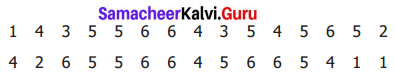Solution:
We prepare a table using tally marks from the given information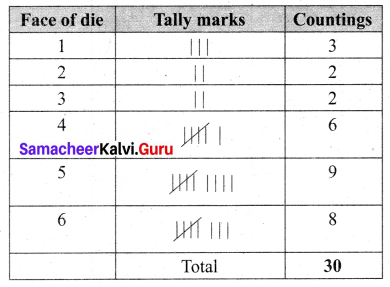Question 3.
The following list tells colours liked by 25 students. Prepare a table using Tally Marks.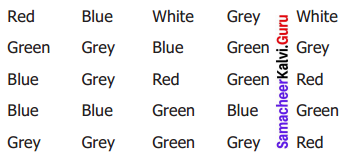Solution:
We prepare the table using Tally marks as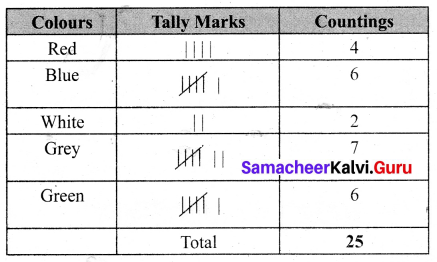Question 4.
The following are the marks obtained by 30 students in a class test out of 20 in Mathematics subject.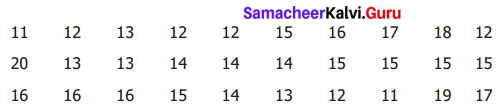Prepare a table using Tally Marks.
Solution:
We prepare a table using Tally Marks as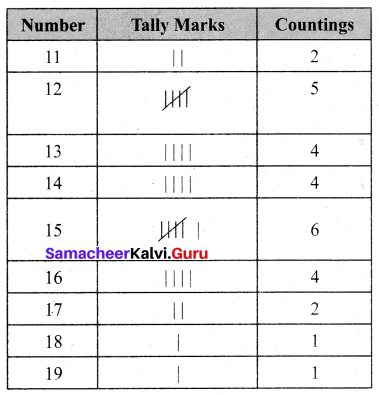Question 5.
The table shows the number of calls recorded by a Fire Service Station in one year.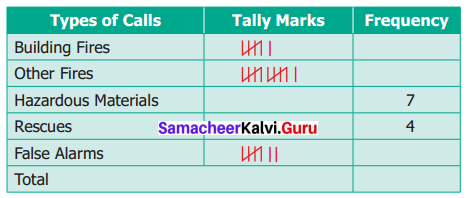Complete the table and answer the following questions.
(i) Which type of call was recorded the most?
(ii) Which type of call was recorded the least?
(iii) How many calls were recorded in all?
(iv) How many calls were recorded as False Alarm?
Solution:
The completed table is given below.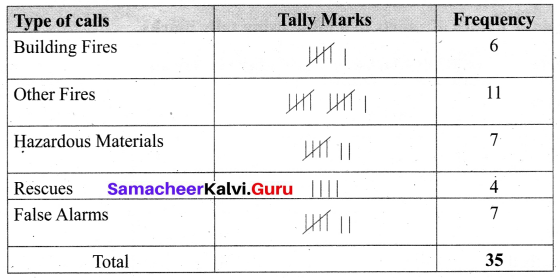(i) The call for “Other Fires” was recorded the most
(ii) The call for “Rescues” was recorded the least
(iii) The total of 35 calls was recorded
(iv) There are 7 calls were recorded as False alarm.Objective Type Questions

Question 6.
The tally marks for the number 7 in standard form is ________
(a) 7
(b)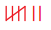(c) ✓✓✓✓✓✓✓
(d)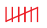Solution:
(b)Question 7.
The tally marks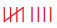represent the number count
(a) 5
(b) 8
(c) 9
(d) 10
Solution:
(c) 9

Question 8.
The plural form of ‘datum’ is
(a) datum
(b) datums
(c) data
(d) datas
Solution:
(c) data